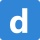Home
IT Knowledge
Inspiration
Languages
EN

# MySQL - get table size (in MB)

0 points
Created by:Dirask Community
6810

In this article, we would like to show you how to get table size in MySQL.

Quick solution:

``````SELECT
TABLE_NAME AS `Table`,
ROUND(((data_length + index_length) / 1024 / 1024), 2) `Size (in MB)`
FROM information_schema.TABLES
WHERE table_schema = "database_name"
AND TABLE_NAME = "table_name";``````

Note:

Replace only the `database_name` and `table_name` from the last 2 lines of code.

## Practical example

Let's say we have the following database:

What interests us will be the size of the `users` table shown in the image above. We want to get it with a query.

Query:

``````SELECT
TABLE_NAME AS `Table`,
ROUND(((data_length + index_length) / 1024 / 1024), 3) `Size (in MB)`
FROM information_schema.TABLES
AND TABLE_NAME = "users";``````

Result: